# DScience:2D representation of data

Limitted access. Login to DataMelt if you are a full DataMelt member.

30% complete

A Plot is a technique for graphical representation of data. Two-dimensional (2D) plots are graph showing the relationship between two variables, X and Y.

# Interactive 2D canvases

Below we discuss canvases which can be used to display data in 2D. Such canvases usually have the names starting with the capital "H".

The code shown below creates a canvas with the size 600x400 (in pixels), it has 2 pads to show data. The method visible(100,200) make the canvas visible and sets its location on the screen at position 100 (in X from left corner) and 200 (from top) in pixels. If you want a default position, jut call "visible()". Then you can draw any mathematical object or data. Then you can export the image to vector format. See thejhplot.HPlot documentation. It should be noted thejhplot.HPlot canvas can be replaced by any other canvas described above.

Typically, you can build a plot to show 2D data as this:

# Exporting to images

When DMelt functions, histograms, data structures are shown, one can create an image using the menu [File]-[Export]. One can also save image in a file using the method "export(file)" to a variety of vector graphics formats as well as bitmap image formats.

In the example below, we save an instance of the HPlot class to a PDF file:

`c1.export('image.pdf')`

we export drawings on the canvas HPlot (c1 represents its instance) into a PDF file. One can also export it into PNG, JPEG, EPS, PS etc. formats using the appropriate extension for the output file name.

As example, simply append the statement "c1.export('image.ps')" at the end of code, and your image will be saved to a PostScript file "image.ps".

Images can be generated in a background (without a pop-up frame with the canvas). For this, use the method "c1.visible(0)", where "0" means Java false.

# Different plot styles

## Line plots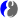Line chart is a chart which displays information as a series of data points called 'markers' connected by straight line segments.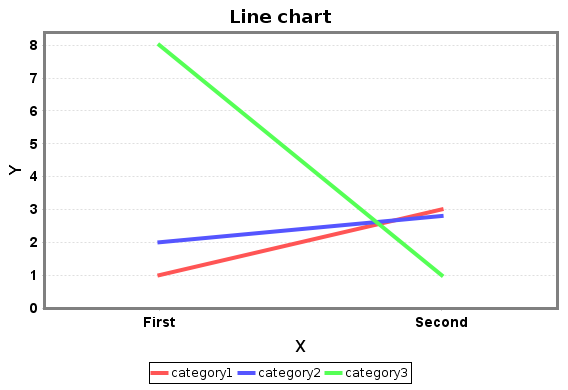with the code

## Histograms

To make a plot of ahistogram, you need to make a histogram which is an object on its own. Typically, you need to create an obejct usingjhplot.H1D. Some examples of how to deal with histograms are considered in Sect. DScience:Histograms. Typiaclly, histograms are used to show some numerical values such that X-axis is numeric.

The output will be shown as: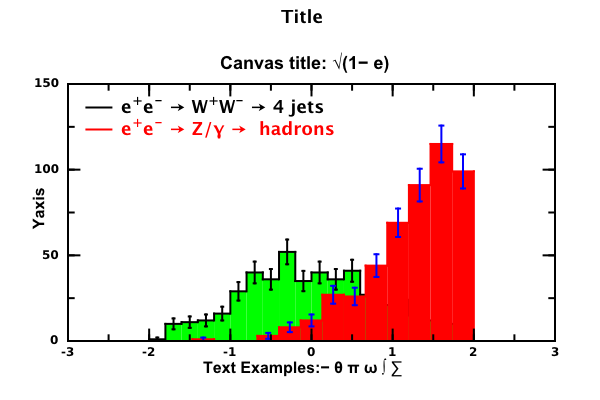## Bar chartsBar chart are similar tohistograms. The bar chart shows categorical data with rectangular bars with heights or lengths proportional to the values that they represent, thus the bar charts are similar to histogram representation of data. The bars can be plotted vertically or horizontally. Thus, the histogram representation is a special case of bar charts.

An example of bar chart is shown here: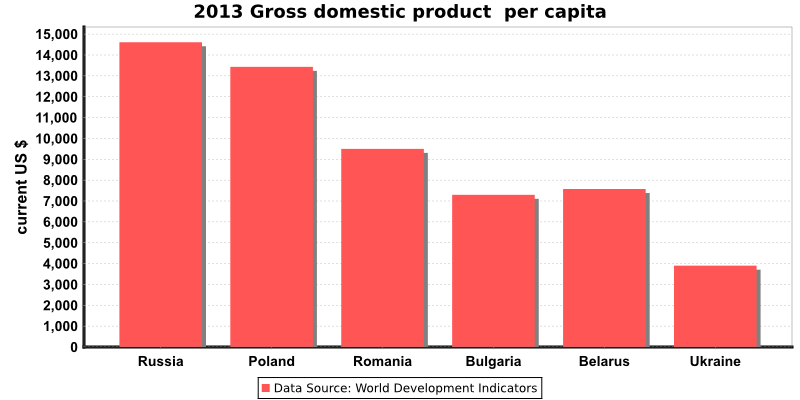## Pie ChartPie chart is a circular gpaph which is divided into slices. The slices illustrate numerical proportion such that the arc length of each slice is proportional to the quantity it represents. It is named for its resemblance to a pie.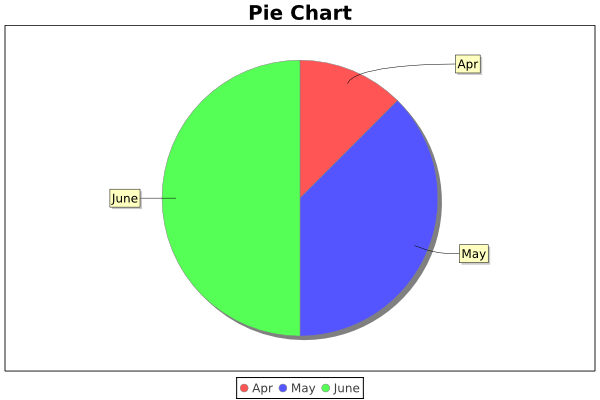```from org.jfree.chart import ChartFactory
from org.jfree.data.general import DefaultPieDataset
from jhplot import HPlotChart

piedataset =DefaultPieDataset()
piedataset.setValue("Apr", 10)
piedataset.setValue("May", 30)
piedataset.setValue("June", 40)

chart = ChartFactory.createPieChart("Pie Chart",piedataset,1,1,1)
c1 = HPlotChart( chart )
c1.visible()```

## Discrete data points

Such plots typically shows position of points in X-Y. In a simple case, they can be made as this: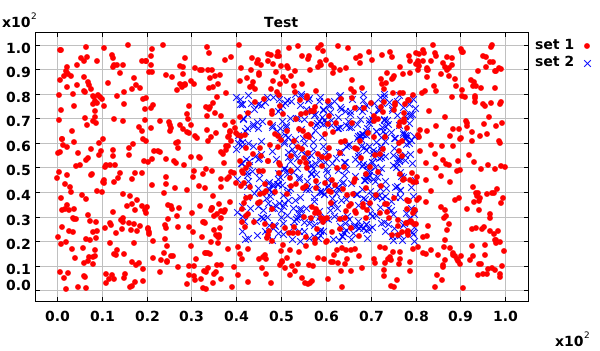Generally, if you need to show error bars in X or Y, usejhplot.P1D data containers (will be discussed later).

## Polar plots

For the polar coordinates, use thejhplot.HChart canvas. A small code below shows ho to show a dataset filled from the X-Y arrayjhplot.P1D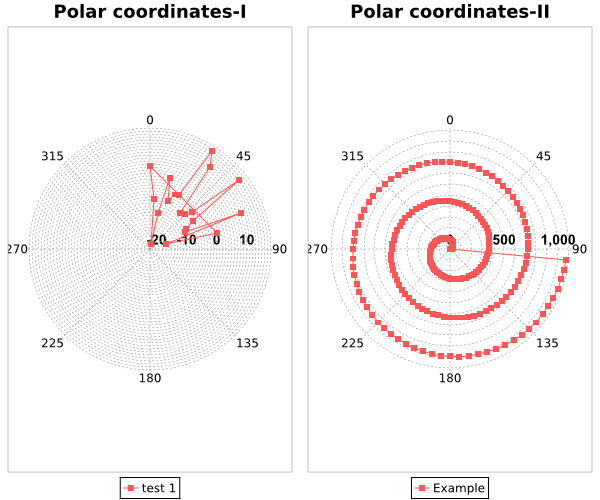## Density plots

You can also make density plots in which color represent density (or values). Look at this rather comprehensive example which shows how to plot F2D functions or 2D histograms (H2D) using several pads: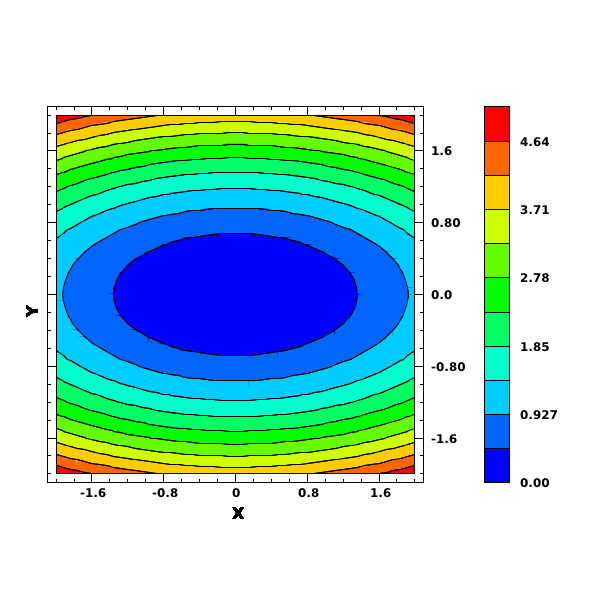## Showing shapes and objects

You can show data and functions together with different 2D objects. Here is a simple example that shows a histogram, a data set in X-Y and 3 ellipses:

Here is the output of this example: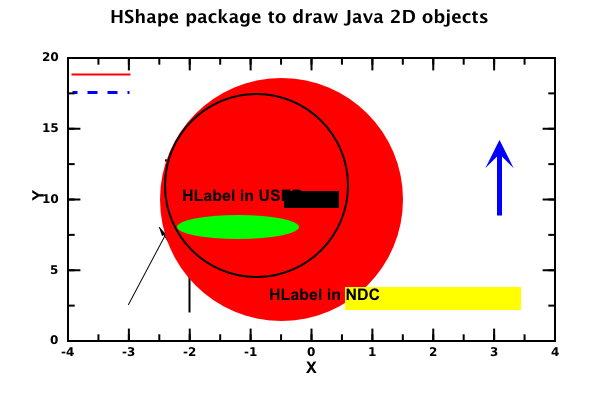# Post editingjhplot.HPlotJa can be used to edit figures. For example, one can make inserts if one creates 2 plotting pads and then one can edit the pads using the "mouse-click" fashion. For example, run this script:

Then edit the figure (increase the size of one pad, and then drag the other one):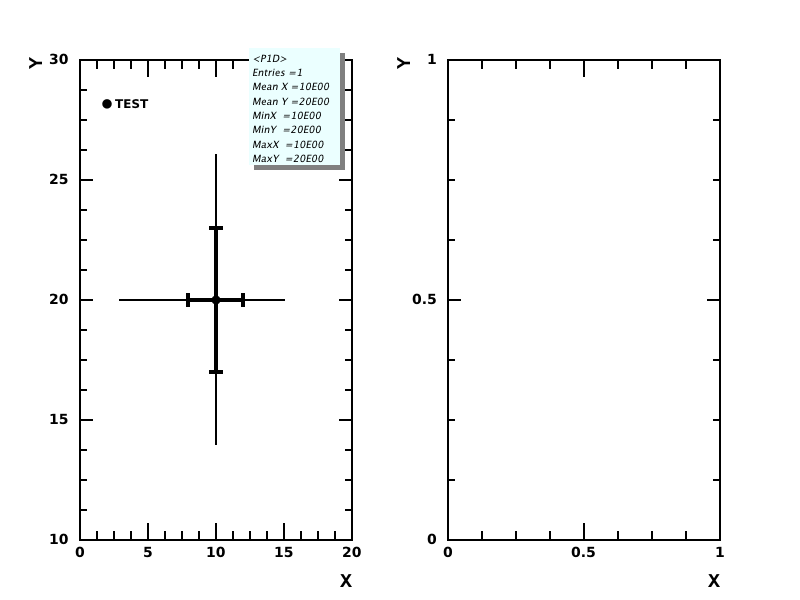Similarly, one can achieve the same using the method "setPad()" where you can specify the location and the sizes of the plot regions The script below creates 2 plotting pads. The second pad is located inside the first one. Then you can plot data as usual, navigating to certain pads using the "cd(i,j)" method.

# Several plotting regions

These canvases can be used to show several pads (plot regions) with plotted objects. Specify the number of divisions in X and Y in the constructors. The navigate to a specific plot region using the method "cd()". Here is example of how to create 2x2 pads on a single canvas:

```from jhplot  import *

c1 = HPlot("Canvas",500,400,2,2)
c1.visible()
c1. setRangeAll(0,10,0,10)
h1 = P1D("Simple")

c1.cd(1,1)

c1.cd(1,2)

c1.cd(2,1)

c1.cd(2,2)
c1.export ("example.pdf") # export to PDF```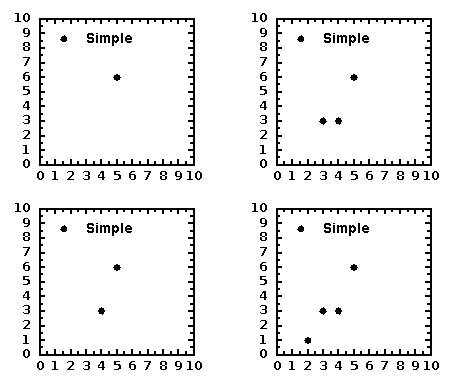Here we use the same X and Y ranges. One can use "setAutoRange()" (after each "cd()") method to set autorange for each pad. Also, try "setRange(xmin,xmax,ymin,ymax)" to set fixed ranges for each pads. it shows 4 pads with data points.

The plots are fully customizable, i.e. one can change any attribute (axis, label, ticks, ticks label).

# Interactive plottingjhplot.HPlotJas canvas represents a way to prepare all objects for plotting, fitting and rebinning of data.

# Axis labels

Can be set using setNameX("label") and setNameY("label"). These are global methods which should be applied to the HPlot canvas. However, every plotting object have their own methods, such as "setLabelX("label")" and setLabelY("label")". If the labels are set to the object, the plot will display the object labels rather than those set using setNameX() and setNameY().

# Ticks and subticks

One can redefine ticks using several methods of thejhplot.HPlot

The simple example illustrates this:

```from jhplot  import *

c1 = HPlot("Canvas")
c1.visible()
c1.setRange(0,10,0,10)
c1.setNumberOfTics(0,2)
c1.setNumberOfTics(1,5)
c1.setSubTicNumber(0,2)
c1.setSubTicNumber(1,4)

h1 = P1D("Simple1")
xpos=5
ypos=7
c1.draw(h1)

lab=HLabel("Point", xpos, ypos, "USER")
c1.update()
c1.export ("example.pdf")```

We labeled a point and generated a PDF files with the figure as shown in this figure: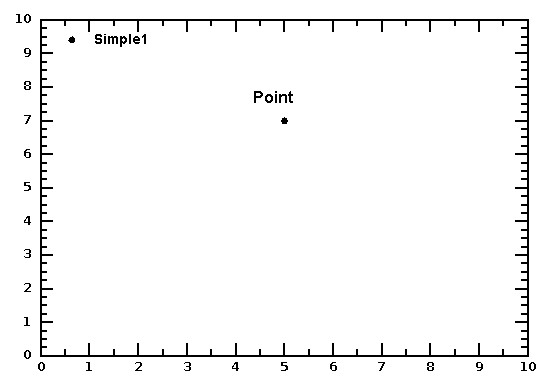# Embedding in JFrame

It is possible to embed DataMelt canvases in Javajava.swing.JFrame, so you can build an application with custom buttons. Here is an example:

# Using GROOT

DMelt includes GROOT package developed at JLab. Its syntax reminds the PyROOT environment. You can find example in Jython and Java here:

# Waterloo scientific charts

DataMelt allows to access reach Waterloo scientific graphics package implemented in Java. The original web page is . However, DataMelt only used the base of the Waterloo package. A support for Groovy and Jython is done via the internal DataMelt api.

The API of these Java libraries for scientific plotting can be foundWaterloo graphics package. Let us give a small example of X-Y plot:

This plot shows the function x*x as shown below: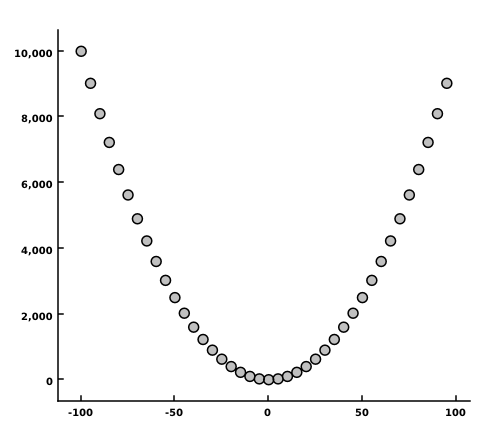This tutorial is provided under this license agreement.